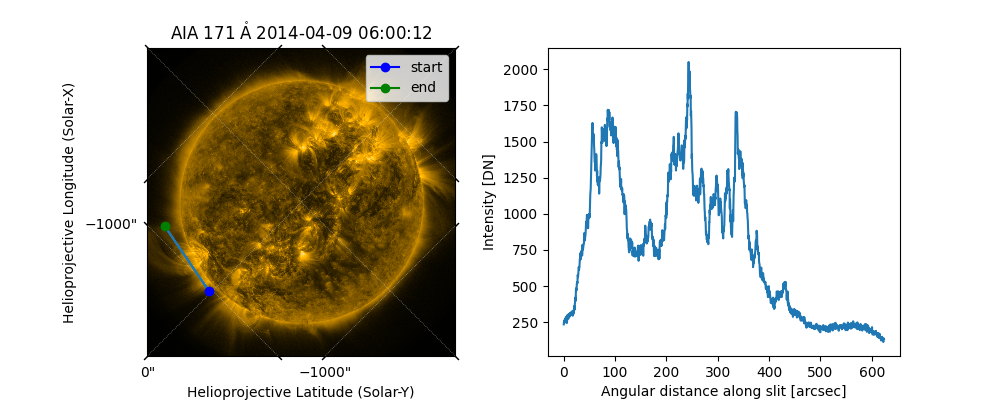# Extracting intensity of a map along a line#

In this example we will extract the intensity values of all the pixels that intersect with a given set of coordinates.

import matplotlib.pyplot as plt

import astropy.units as u
from astropy.coordinates import SkyCoord

import sunpy.map
from sunpy.data.sample import AIA_171_ROLL_IMAGE


First we construct a map, using some sample data.

aia_map = sunpy.map.Map(AIA_171_ROLL_IMAGE)


Next we define a path in a SkyCoord object. In this example we are just going to use a straight line defined by two points. However a path with any number of points and any shape can be used.

line_coords = SkyCoord([-1024, -908], [20, 633], unit=(u.arcsec, u.arcsec),
frame=aia_map.coordinate_frame)


Next we call the sunpy.map.pixelate_coord_path() function with the map and the coordinate path to obtain the coordinates of the map pixels that intersect that path. We pass those coordinates to sunpy.map.sample_at_coords() to extract the values for those map pixels.

intensity_coords = sunpy.map.pixelate_coord_path(aia_map, line_coords)
intensity = sunpy.map.sample_at_coords(aia_map, intensity_coords)


Next we will calculate the angular separation between the first point and every other coordinate we extracted. We are doing this to give us a meaningful x-axis for our line plot below.

angular_separation = intensity_coords.separation(intensity_coords).to(u.arcsec)


Finally let’s plot the results.

fig = plt.figure(figsize=(10, 4))
aia_map.plot(axes=ax1)
ax1.plot_coord(intensity_coords)
ax1.plot_coord(line_coords, marker="o", color="blue", label="start")
ax1.plot_coord(line_coords, marker="o", color="green", label="end")
ax1.legend()